Minima/ maxima (statement questions) - Geometry questions

Chapter 6 Class 12 Application of Derivatives
Concept wise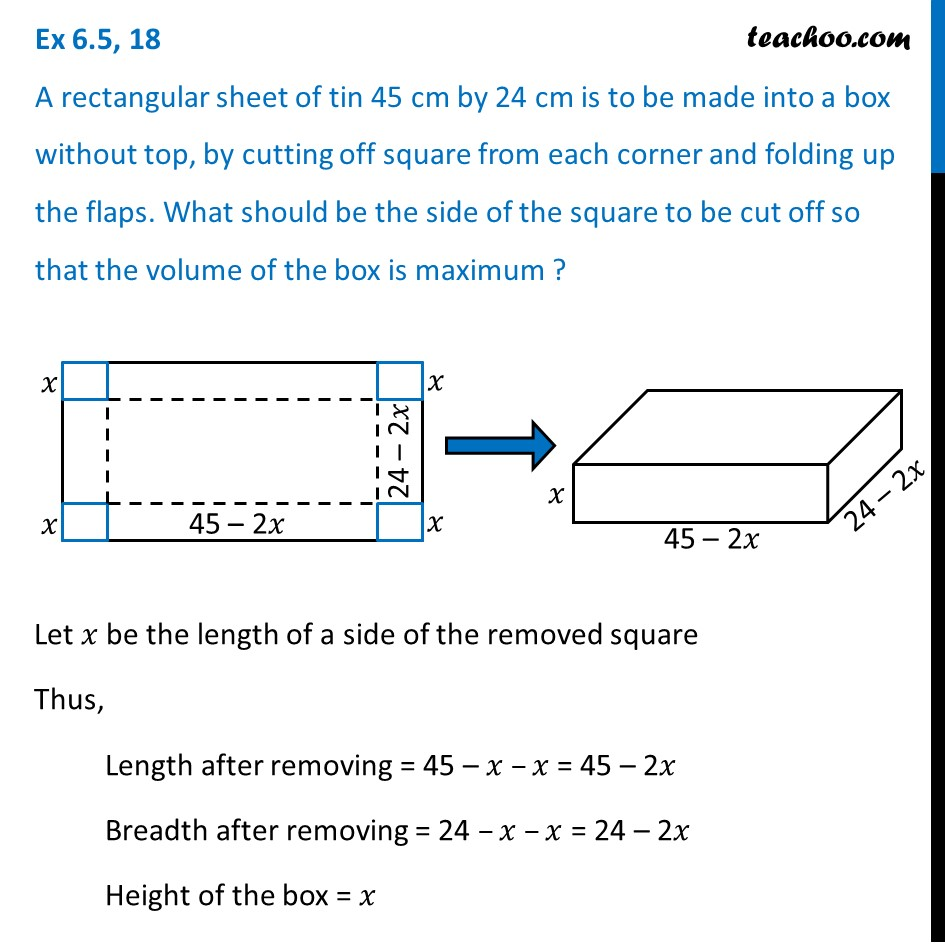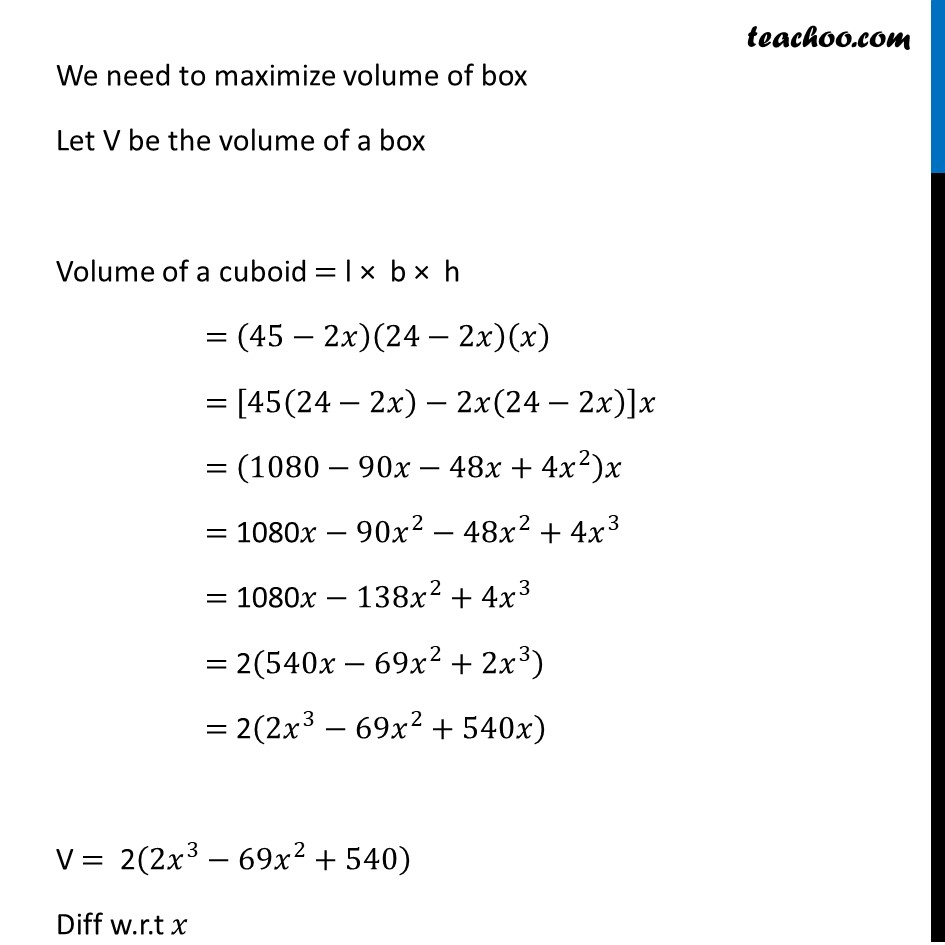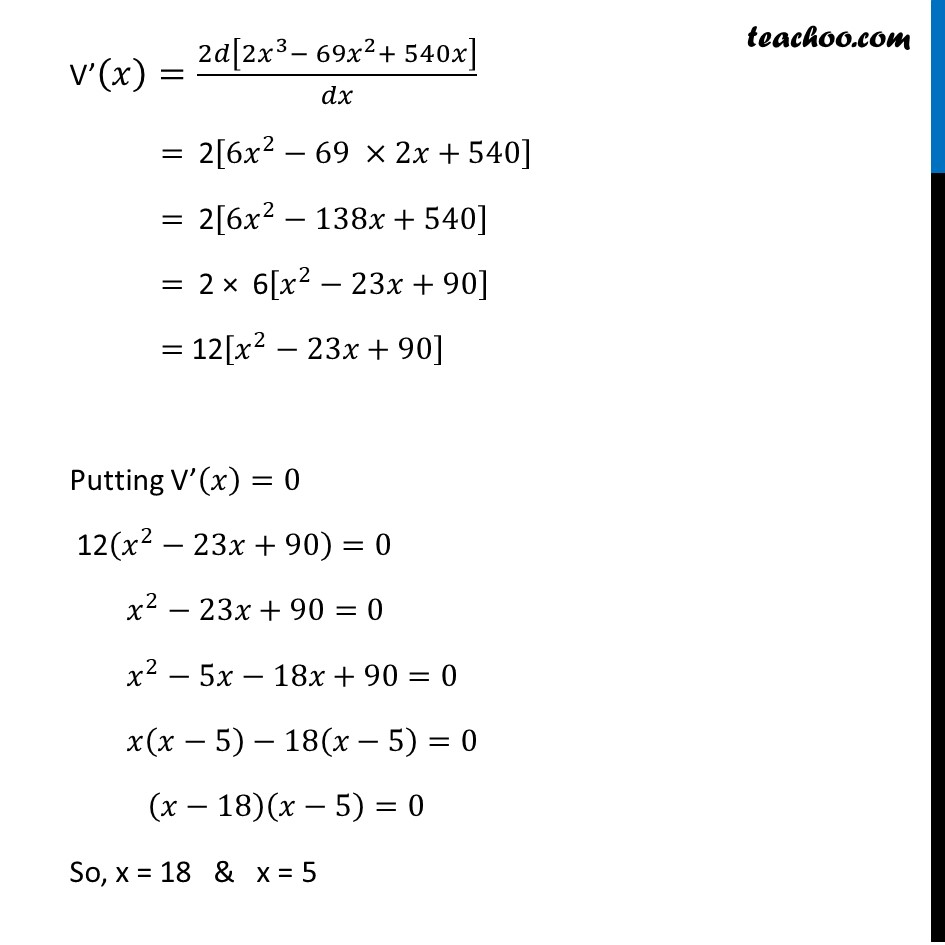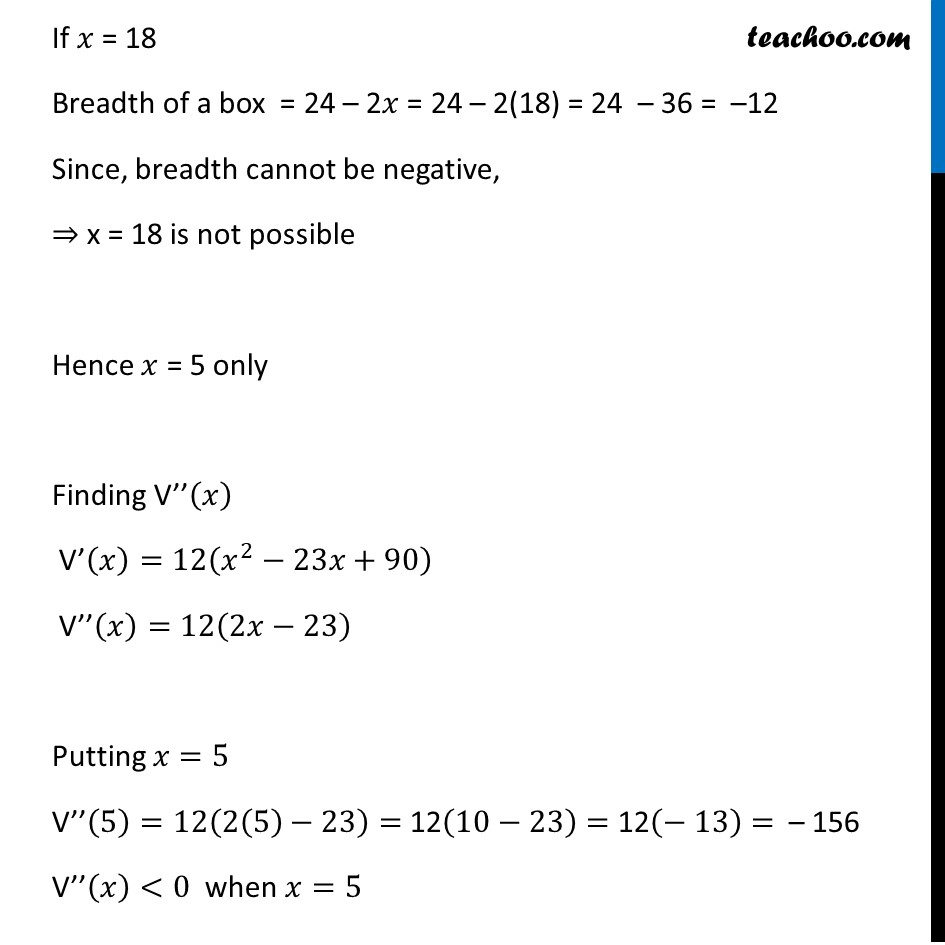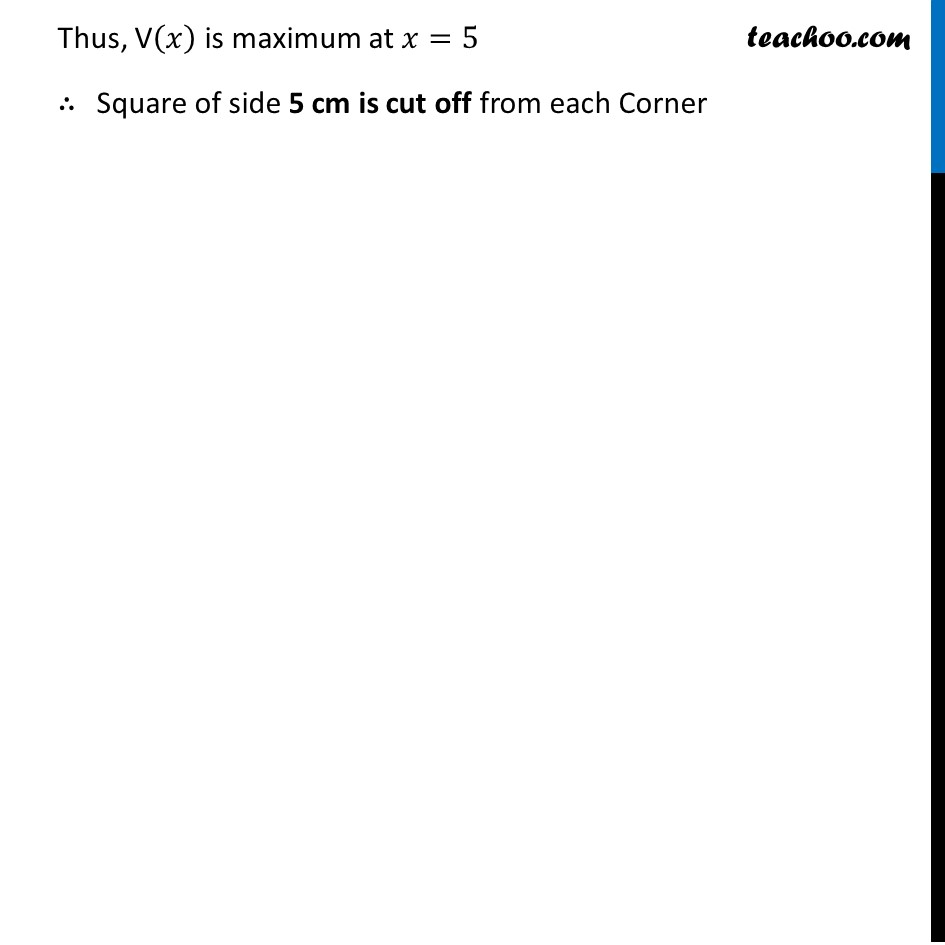Introducing your new favourite teacher - Teachoo Black, at only ₹83 per month

### Transcript

Ex 6.5, 18 A rectangular sheet of tin 45 cm by 24 cm is to be made into a box without top, by cutting off square from each corner and folding up the flaps. What should be the side of the square to be cut off so that the volume of the box is maximum ?Let 𝑥 be the length of a side of the removed square Thus, Length after removing = 45 – 𝑥 –𝑥 = 45 – 2𝑥 Breadth after removing = 24 –𝑥 –𝑥 = 24 – 2𝑥 Height of the box = 𝑥 We need to maximize volume of box Let V be the volume of a box Volume of a cuboid = l × b × h = (45−2𝑥)(24−2𝑥)(𝑥) = [45(24−2𝑥)−2𝑥(24−2𝑥)]𝑥 = (1080−90𝑥−48𝑥+4𝑥^2 )𝑥 = 1080𝑥−90𝑥^2−48𝑥^2+4𝑥^3 = 1080𝑥−138𝑥^2+4𝑥^3 = 2(540𝑥−69𝑥^2+2𝑥^3 ) = 2(2𝑥^3−69𝑥^2+540𝑥) V = 2(2𝑥^3−69𝑥^2+540) Diff w.r.t 𝑥 V’(𝑥)=2𝑑[2𝑥^3− 69𝑥^2+ 540𝑥]/𝑑𝑥 = 2[6𝑥^2−69 ×2𝑥+540] = 2[6𝑥^2−138𝑥+540] = 2 × 6[𝑥^2−23𝑥+90] = 12[𝑥^2−23𝑥+90] Putting V’(𝑥)=0 12(𝑥^2−23𝑥+90)=0 𝑥^2−23𝑥+90=0 𝑥^2−5𝑥−18𝑥+90=0 𝑥(𝑥−5)−18(𝑥−5)=0 (𝑥−18)(𝑥−5)=0 So, x = 18 & x = 5 If 𝑥 = 18 Breadth of a box = 24 – 2𝑥 = 24 – 2(18) = 24 – 36 = –12 Since, breadth cannot be negative, ⇒ x = 18 is not possible Hence 𝑥 = 5 only Finding V’’(𝑥) V’(𝑥)=12(𝑥^2−23𝑥+90) V’’(𝑥)=12(2𝑥−23) Putting 𝑥=5 V’’(5)=12(2(5)−23)= 12(10−23)= 12(− 13)= – 156 V’’(𝑥)<0 when 𝑥=5 Thus, V(𝑥) is maximum at 𝑥=5 ∴ Square of side 5 cm is cut off from each Corner